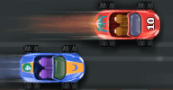Empowered Learner### Overview​

This Turtle Diary game helps students practice addition through solving addition problems through a car racing game. This game is a great option for independent center practice, whole group instruction, or small group instruction. The game format is slow, provides explicit explanations for students to grasp, and provides differentiation through a variety of levels with increased difficulty. Students are given four equations and asked to find the correct addition equation. If students choose an incorrect equation, the equation becomes red. When students choose a correct equation, the equation becomes green. When students answer incorrectly, their race car slows down. For each correct answer, their race car will speed up. The goal of this game is to answer the most correct equations and pass the finish line first.

Students will:

• Be able to identify the addition symbol.
• Be able to solve addition equations.
• Be able to select the correct answer for the equations.

Vocabulary Words:

1. Select: To select is choosing the most appropriate choice.
2. Equation: An equation is a statement where the values of two mathematical expressions are equal (indicated by the sign =)
3. Speed Up: To speed up is an increase of speed.
4. Slow Down: To slow down is an act of slowing down.
5. Finish: Finish is the end of something.

To prepare for this lesson:

• Visit the Turtle Diary website

• Teachers should have previously taught mathematical symbols such as + and =.

• Teachers should have previously demonstrated the “putting together” and “taking away” process.

• Teachers should have previously shown equations both vertically and horizontally (the equations in this game are presented horizontally).

See Accommodations Page and Charts on the 21things4students.net site in the Teacher Resources.

Teacher Directions for this activity:

1. Have the students begin the activity on Turtle Diary.
2. Provide whiteboard and expo markers if students need more of a visual or tactile process.
3. Make sure your computer speakers or headphones are connected for the best game quality.

Student Directions for this activity:

2. Select the math equation that is correct.
3. Continue to read and choose math equations until your car crosses the finish line.

Different options for assessing the students:

• Observations
• Check for understanding
• Ask students to teach one another the process of reading the math equations.
• Data Collection for baseline and growth.

MITECS: Michigan adopted the "ISTE Standards for Students" called MITECS (Michigan Integrated Technology Competencies for Students) in 2018.

Empowered Learner
1a. Students develop learning goals in collaboration with an educator, select the technology tools to achieve them, and revise the learning process as needed to achieve the goal.
1c. Students use technology to seek feedback that informs and improves their practice and to demonstrate their learning in a variety of ways.

Browser: Chrome, Safari, Firefox, Edge, ALL